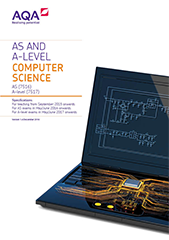# 3.1 Fundamentals of programming

## Programming

### Data types

Content

Understand the concept of a data type.

Understand and use the following appropriately:

• integer
• real/float
• Boolean
• character
• string
• date/time
• records (or equivalent)
• arrays (or equivalent).

Define and use user-defined data types based on language-defined (built-in) data types.

### Programming concepts

Content

Use, understand and know how the following statement types can be combined in programs:

• variable declaration
• constant declaration
• assignment
• iteration
• selection
• subroutine (procedure/function).

The three combining principles (sequence, iteration/repetition and selection/choice) are basic to all imperative programming languages.

Use definite and indefinite iteration, including indefinite iteration with the condition(s) at the start or the end of the iterative structure. A theoretical understanding of condition(s) at either end of an iterative structure is required, regardless of whether they are supported by the language being used.

Use nested selection and nested iteration structures.

Use meaningful identifier names and know why it is important to use them.

### Arithmetic operations in a programming language

Content

Be familiar with and be able to use:

• subtraction
• multiplication
• real/float division
• integer division, including remainders
• exponentiation
• rounding
• truncation.

### Relational operations in a programming language

Content

Be familiar with and be able to use:

• equal to
• not equal to
• less than
• greater than
• less than or equal to
• greater than or equal to.

### Boolean operations in a programming language

Content

Be familiar with and be able to use:

• NOT
• AND
• OR
• XOR.

### Constants and variables in a programming language

Content

Be able to explain the differences between a variable and a constant.

Be able to explain the advantages of using named constants.

### String-handling operations in a programming language

Content

Be familiar with and be able to use:

• length
• position
• substring
• concatenation
• character → character code
• character code → character
• string conversion operations.

Expected string conversion operations:

• string to integer
• string to float
• integer to string
• float to string
• date/time to string
• string to date/time.

### Random number generation in a programming language

Content

Be familiar with, and be able to use, random number generation.

### Exception handling

Content

Be familiar with the concept of exception handling.

Know how to use exception handling in a programming language with which students are familiar.

### Subroutines (procedures/functions)

Content

Be familiar with subroutines and their uses.

Know that a subroutine is a named ‘out of line’ block of code that may be executed (called) by simply writing its name in a program statement.

Be able to explain the advantages of using subroutines in programs.

### Parameters of subroutines

Content

Be able to describe the use of parameters to pass data within programs.

Be able to use subroutines with interfaces.

### Returning a value/values from a subroutine

Content

Be able to use subroutines that return values to the calling routine.

### Local variables in subroutines

Content

Know that subroutines may declare their own variables, called local variables, and that local variables:

• exist only while the subroutine is executing
• are accessible only within the subroutine.

Be able to use local variables and explain why it is good practice to do so.

### Global variables in a programming language

Content

Be able to contrast local variables with global variables.

## Procedural-oriented programming

### Structured programming

Content